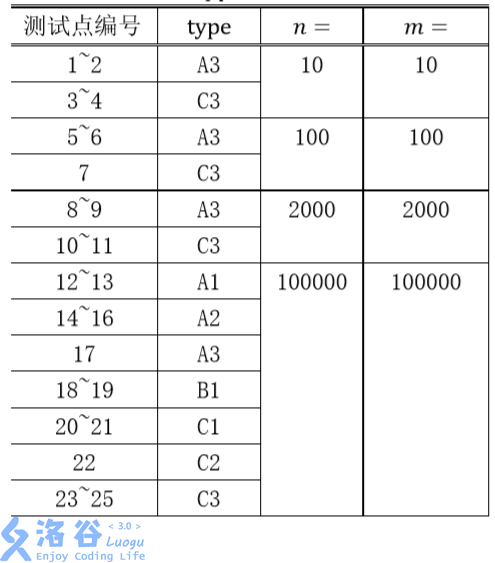动态DP

保卫王国

Description

Z国有$n$ 座城市，$n-1$ 条双向道路，每条双向道路连接两座城市，且任意两座城市

Z国的国防部长小Z要在城市中驻扎军队。驻扎军队需要满足如下几个条件：
● 一座城市可以驻扎一支军队，也可以不驻扎军队。
● 由道路直接连接的两座城市中至少要有一座城市驻扎军队。
● 在城市里驻扎军队会产生花费，在编号为$i$的城市中驻扎军队的花费是$p_i$ 。

Hint

【样例解释】

【数据规模与约定】$A$：城市ii与城市$i +1$直接相连。
$B$：任意城市与城市 $1$ 的距离不超过 $100$（距离定义为最短路径上边的数量），即如果这棵树以 $1$ 号城市为根，深度不超过 $100$。
$C$：在树的形态上无特殊约束。
$1$：询问时保证$a = 1,x = 1$即要求在城市 $1$ 驻军。对$b,y$没有限制。
$2$：询问时保证$a,b$是相邻的（由一条道路直接连通）
$3$：在询问上无特殊约束。

洛谷p4751

Hint

$n\leq1∗10^6$,$m \leq 3*10^6$Solution

（这难度咋跟上一道一样啊=.=可能是没有更高的了吧？？？）然而你谷日常入门难度打NOI+

BZOJ4712

Input

$n<=200000$，保证任意$to$都为非负数

BZOJ5210

Description

$M\ x\ y$：将点$x$的点权改为$y$；
$Q\ x$：求以$x$为根的子树的最大连通子块和。

（本题中子连通块包括空连通块，点权和为$0$）。

Input

$1≤n,m≤200000$，任意时刻$|w_i|≤10^9$ 。

Solution

（并不是）一眼得出转移式子$f[u]=max(0,\sum{f[v]}+w[u])$